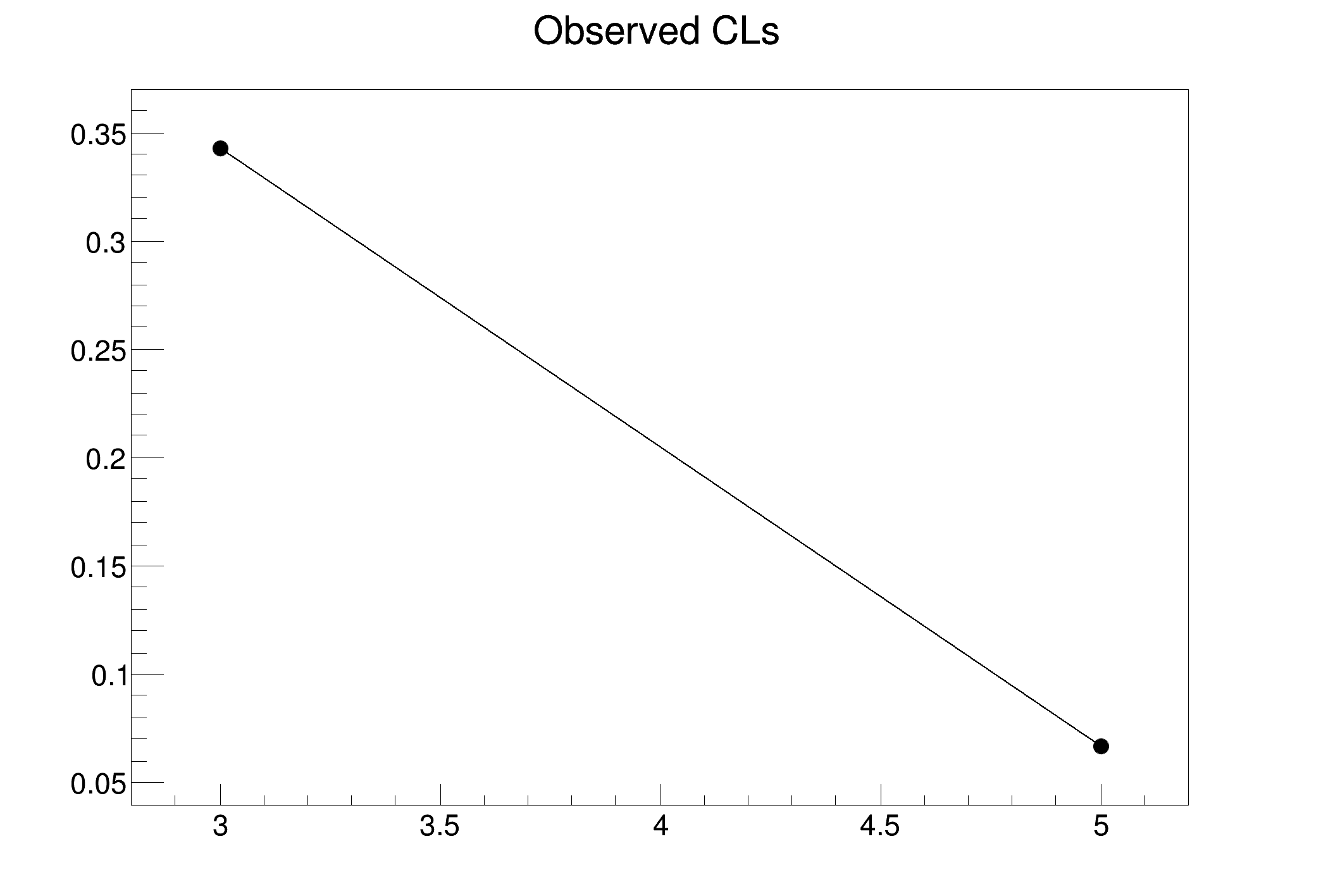ROOT   Reference Guiders801_HypoTestInverterOriginal.C File Reference

## Detailed DescriptionAn example of using the HypoTestInverterOriginal class␛[1mRooFit v3.60 -- Developed by Wouter Verkerke and David Kirkby␛[0m
Copyright (C) 2000-2013 NIKHEF, University of California & Stanford University
Target matches upper limit: de-activate interpolation in HypoTestInverterResult
Running for r = 3
Test statistics has been evaluated for data
HybridCalculatorOriginal: run 1000 toy-MC experiments
with test statistics index: 2
....... toy number 0 / 1000
....... toy number 500 / 1000
computed: 0.34252
Running for r = 5
Test statistics has been evaluated for data
HybridCalculatorOriginal: run 1000 toy-MC experiments
with test statistics index: 2
....... toy number 0 / 1000
....... toy number 500 / 1000
computed: 0.0669145
The confidence level at both boundaries are both too large ( 0.34252 and 0.0669145
Run again with other boundaries or larger toy-MC statistics
The computed upper limit is: 5
an estimated error on this upper limit is: 0
#include "RooRealVar.h"
#include "RooConstVar.h"
#include "RooProdPdf.h"
#include "RooWorkspace.h"
#include "RooDataSet.h"
#include "RooPolynomial.h"
#include "RooExtendPdf.h"
#include "TGraphErrors.h"
using namespace RooFit;
using namespace RooStats;
void rs801_HypoTestInverterOriginal()
{
// prepare the model
RooRealVar lumi("lumi", "luminosity", 1);
RooRealVar r("r", "cross-section ratio", 3.74, 0, 50);
RooFormulaVar ns("ns", "1*r*lumi", RooArgList(lumi, r));
RooRealVar nb("nb", "background yield", 1);
RooRealVar x("x", "dummy observable", 0, 1);
RooPolynomial flatPdf("flatPdf", "flat PDF", x, p0);
RooAddPdf totPdf("totPdf", "S+B model", RooArgList(flatPdf, flatPdf), RooArgList(ns, nb));
RooExtendPdf bkgPdf("bkgPdf", "B-only model", flatPdf, nb);
RooDataSet *data = totPdf.generate(x, 1);
// prepare the calculator
HybridCalculatorOriginal myhc(*data, totPdf, bkgPdf, 0, 0);
myhc.SetTestStatistic(2);
myhc.SetNumberOfToys(1000);
myhc.UseNuisance(false);
// run the hypothesis-test inversion
HypoTestInverterOriginal myInverter(myhc, r);
myInverter.SetTestSize(0.10);
myInverter.UseCLs(true);
// myInverter.RunFixedScan(5,1,6);
// scan for a 95% UL
myInverter.RunAutoScan(3., 5, myInverter.Size() / 2, 0.005);
// run an alternative autoscan algorithm
// myInverter.RunAutoScan(1,6,myInverter.Size()/2,0.005,1);
// myInverter.RunOnePoint(3.9);
HypoTestInverterResult *results = myInverter.GetInterval();
HypoTestInverterPlot myInverterPlot("myInverterPlot", "", results);
TGraphErrors *gr1 = myInverterPlot.MakePlot();
gr1->Draw("ALP");
double ulError = results->UpperLimitEstimatedError();
double upperLimit = results->UpperLimit();
std::cout << "The computed upper limit is: " << upperLimit << std::endl;
std::cout << "an estimated error on this upper limit is: " << ulError << std::endl;
// expected result: 4.10
}
int main()
{
rs801_HypoTestInverterOriginal();
}
ROOT::R::TRInterface & r
Definition: Object.C:4
RooAddPdf is an efficient implementation of a sum of PDFs of the form.
RooArgList is a container object that can hold multiple RooAbsArg objects.
Definition: RooArgList.h:21
RooConstVar represent a constant real-valued object.
Definition: RooConstVar.h:25
RooDataSet is a container class to hold unbinned data.
Definition: RooDataSet.h:33
RooExtendPdf is a wrapper around an existing PDF that adds a parameteric extended likelihood term to ...
Definition: RooExtendPdf.h:22
A RooFormulaVar is a generic implementation of a real-valued object, which takes a RooArgList of serv...
Definition: RooFormulaVar.h:29
RooPolynomial implements a polynomial p.d.f of the form.
Definition: RooPolynomial.h:28
RooRealVar represents a variable that can be changed from the outside.
Definition: RooRealVar.h:35
A TGraphErrors is a TGraph with error bars.
Definition: TGraphErrors.h:26
virtual void Draw(Option_t *chopt="")
Draw this graph with its current attributes.
Definition: TGraph.cxx:760
RooConstVar & RooConst(Double_t val)
int main(int argc, char **argv)
Double_t x[n]
Definition: legend1.C:17
The namespace RooFit contains mostly switches that change the behaviour of functions of PDFs (or othe...
Namespace for the RooStats classes.
Definition: Asimov.h:19
static constexpr double ns

Definition in file rs801_HypoTestInverterOriginal.C.